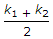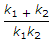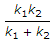# Mechanical Engineering - Strength of Materials

### Exercise :: Strength of Materials - Section 1

36.

In order to know whether a column is long or short, we must know its slenderness ratio.

 A. True B. False

Explanation:

No answer description available for this question. Let us discuss.

37.

A composite shaft consisting of two stepped portions having spring constants k1 and k2 is held between two rigid supports at the ends. Its equivalent spring constant is

 A.B.C.D. k1+k2

Explanation:

No answer description available for this question. Let us discuss.

38.

Resilience is the

 A. energy stored in a body when strained within elastic limits B. energy stored in a body when strained upto the breaking of the specimen C. maximum strain energy which can be stored in a body D. none of the above

Explanation:

No answer description available for this question. Let us discuss.

39.

If the depth is kept constant for a beam of uniform strength, then its width will vary in proportional to (where M = Bending moment)

 A. M B. M C. M2 D. M3

Explanation:

No answer description available for this question. Let us discuss.

40.

A concentrated load is one which

 A. acts at a point on a beam B. spreads non-uniformly over the whole length of a beam C. spreads uniformly over the whole length of a beam D. varies uniformly over the whole length of a beam